# Texas Go Math Grade 3 Lesson 4.6 Answer Key Money Amounts

Refer to our Texas Go Math Grade 3 Answer Key Pdf to score good marks in the exams. Test yourself by practicing the problems from Texas Go Math Grade 3 Lesson 4.6 Answer Key Money Amounts.

## Texas Go Math Grade 3 Lesson 4.6 Answer Key Money Amounts

Unlock the Problem

Materials; play money for coins and bills

Amanda has some money in her pocket. The money is shown below. How much money does she have?

Circle the question.
Answer: $13. Explanation: As shown below the total money is$13.

Find the amount of money.

STEP 1:
Find the value of each type of bill.There is _______ $10 bill. That is worth$ _______.
There are _______ $1 bills. That is worth$ _______.
The value of the bills is $_______ +$_______ = $_______. Answer: There is 1$10 bill. That is worth $10 There are 3$1 bills. That is worth $3 The value of the bills is$10 + $3 =$13.
Explanation:
Bill is a piece of paper money worth a specified amount like ten dollar bill.
It is a lowest value denomination.

STEP 2:
Find the value of each type of coin.There are _______ quarter. That is worth $_______. There are _______ dimes. That is worth$ _______.
There are _______ pennies. That is worth $_______. The value of the bills is$_______ + $_______ +$_________ = $_______. Answer: There are 1 quarter. That is worth$ 0.25.
There are 2 dimes. That is worth $0.20. There are 3 pennies. That is worth$0.03.
The value of the bills is $0.25+$0.20+ $0.03=$0.48.
Explanation:
As we know that,
1 quarter = 25 cents or $0.25 1 dime = 10 cents or$0.10
1 penny = 1 cent or $0.01 Remember Start with the bill or coin of the greatest value. STEP 3: Add the values of the bills and coins in Amanda’s pocket.Think: The decimal point separates the dollars from the cents. So, Amanda has$__________ in her packet.
Answer: $13.48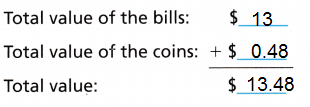Explanation: According to the above information Amanda has,$13 bills and $0.48 coins Total amount he has =$13.48

Share and Show

Find the amount of money.

Question 1.Answer: $12.21 Explanation: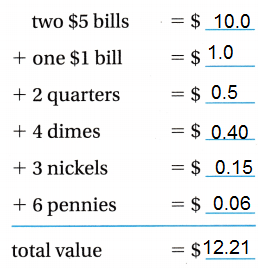Question 2.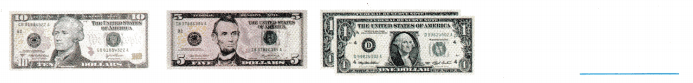Answer:$17
Explanation:
one $10 bill one$5 bill
two $1 bills Total bis together 10 + 5 + 2 = 17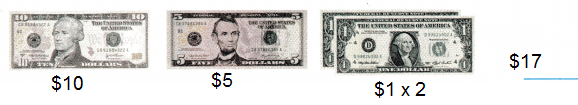Question 3.Answer:$2.54
Explanation: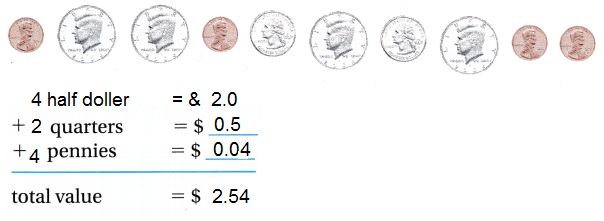Problem Solving

Write the value of the bills or coins.

Question 4.Answer: $20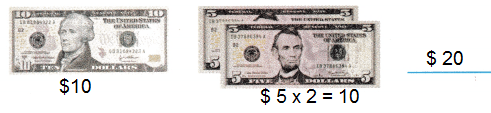Explanation: one$10 bill = $10 two$5 bill = 5 X 2 = $10 Total bills together =$10 + $10 =$20

Question 5.Answer: $1.5Explanation: 1 quarter = 25 cents or$0.25
1 dime = 10 cents or $0.10 1 penny = 1 cent or$0.01
Total amount all together
$0.25 X 5 +$0.10 X 2 + $0,01 X 5$1.25 + $0.20 +$0.05 = $1.5 Question 6. H.O.T. Daniel has one$10 bill, five $1 bills, 3 quarters, I dime, and 3 nickels. How much money does Daniel have? Answer:$11.40
Explanation:
Daniel has one $10 bill, five$1 bills,
3 quarters,
I dime and
3 nickels.
Total amount Daniel have
1 x $10 + 5 x$1 +  3 x $0.25 + 1 x$ 0.10 + 3 x 0.05 = $11.40 Math Talk Mathematical Processes Explain what happens when you have more than 99 cents in a group of coins. Answer: count will start from$1 then cents.
So, 100 cents are convert in to $1. Explanation: The answer is 10 coins, 3 Quarters, 1 dime, 2 nickel, and 4 pennies. With this combination one can produce any number between 1-99 cents. Combination of coins may vary. Problem Solving Question 7. H.O.T. Multi-Step Erin has only quarters. She has more than 75e. Which amount could Erin have?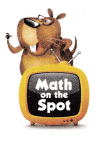(A)$0.50
(B) $0.95 (C)$1.15
(D) $1.25 Answer: Option (D) 0.25 + .025 + .025 + .025 +.025 =1.25 Explanation: if he is has only 0.25, he must have 4 coins or 5 coins of 0.25 cents 4 x 0.25 =$1
or
5 x 0.25 = $1.25 a. What do you need to find? Answer: Total amount Erin has. b. What information will you use to solve the problem? Answer: Erin contains only quarters coins c. Show the steps you used to solve the problem. Answer:$1.25
0.25 + .025 + .025 + .025 +.025 =1.25
Explanation:
if he is has only 0.25, he must have 4 coins or 5 coins of 0.25 cents
4 x 0.25 =$1 or 5 x 0.25 =$1.25

Question 8.
H.O.T. Multi-Step Charlie earned the bills and coins below at his lemonade stand.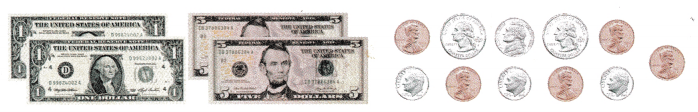Does Charlie have enough money to buy inure lemonade for $8.00? How much nwIìey will he have left? Explain. Answer:$5.10
Explanation:
Charlie has $8.00 Charlie has the following bills and coins$1 x 2 bills =    $2 +$5 x 2 bills=  $10 + 0.01 x 5 pennies =$ 0.25
+  0.10 x 3 dimes = $0.30 + 0.25 x 2 quarters =$ 0.5
+ 0.05 x 1 nickel = $0.05 Total amount = 13.10$13.10 – $8.00 =$5.10

Fill in the bubble for the correct answer choice.

Question 9.
Representations Alejandro saved the money shown. How much did he save?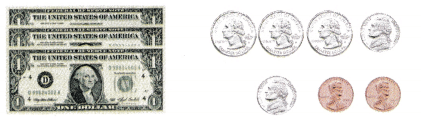(A) $1.62 (B)$2.76
(C) $3.87 (D)$3.97
Option (D)
Explanation:
Representation of Alejandro money is as follows
$1 x 3 bills =$ 3
+  0.01 x 2 pennies =   $0.02 + 0.10 x 2 dimes =$ 0.20
+ 0.25 x 3 quarters = $0.75 Total amount =$3.97
Question 10.
Multi-Step Kara has exactly enough money to buy the puzzle. She has one $5 bill, two$1 bills, and seven coins. What coins does she have?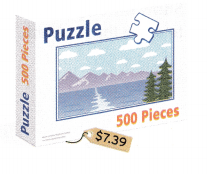(A) 3 dimes and 4 pennies
(B) 2 dimes, 1 nickel, and 4 pennies
(C) 1 quarter, 2 nickels, and 4 pennies
(D) 1 quarter, 1 dime, 1 nickel, and 4 pennies
Option (C)
Explanation:
Kara has one $5 bill, two$1 bills, and seven coins
$5 +$2 = $7 7 coins rest coins as follows 1 quarter, 2 nickel, and 4 pennies 0.25 + 0.10 + 0.04 Texas Test Prep Question 11. What amount of money is shown below?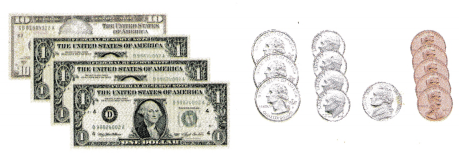(A)$14.50
(B) $14.25 (C)$13.30
(D) $14.10 Answer: Option (B) Explanation: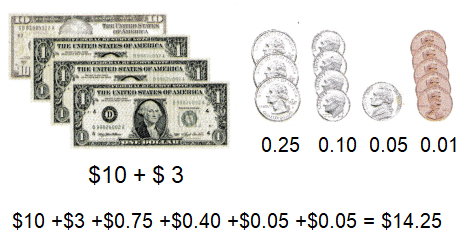### Texas Go Math Grade 3 Lesson 4.6 Homework and Practice Answer Key Find the amount of money. Question 1.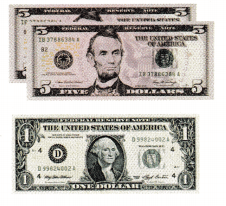Answer:$11
Explanation:
$5 x 2 bills +$1 = $11 Question 2.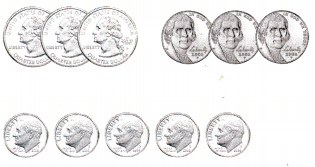Answer:$1.40
Explanation:
$0.25 x 3 quarters +$0.05 x 3 nickels + $0.10 x 5 dimes$0.75 + $0.15 +$0.50 = $1.40 Question 3.Answer:$6.03
Explanation:
$5 +$0.25 x 4 quarters + $0.01 x 3 pennies =$5 + $1 +$0.03
= $6.03 Question 4.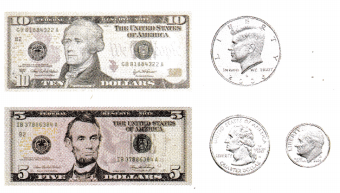Answer:$15.85
Explanation:
$10 +$5 + $0.50 nickel +$0.25 quarter + $0.10 dime =$15.85

Problem Solving

Question 5.
Ally has one $5 bill and two$1 bills. How much money does Ally have?
Answer: $7 Explanation: Ally has one$5 bill and two $1 bills. Total money Ally has 5+1+1 =$7

Question 6.
A cashier gives a customer change. She gives one quarter, 1 dime, and 3 pennies. What is the value of the change?
Answer: $0.38 Explanation: A cashier gives a customer change of one quarter, 1 dime, and 3 pennies. Total value of the change$0.25 + $0.10 + 3 x$0.01 = $0.38 Lesson Check Fill in the bubble completely to show your answer. Question 7. Hal has only quarters. He has less than$1.00. Which amount could Hal have?
(A) 45¢
(B) 65¢
(C) $1.25 (D) 75¢ Answer: Option (D) Explanation: he has only quarters =$0.25
less then $1 , it means he must have 3 x$0.25 = 75¢

Question 8.
Tim gets change in all dimes. He gets less than 50¢. Which amount could Tim get for change?
(A) 60¢
(B) 35 ¢
(C) 40 ¢
(D) 25 ¢
Option (C)
Explanation:
Tim gets change in all dimes less than 50¢.
Total amount Tim got for change 4 dimes
1 dime = 10 cent
So, 10 x 4 = 40 cents.

Question 9.
Dave spent the amount of money shown. How much did he spend?(A) $3.48 (B)$3.23
(C) $3.47 (D)$2.48
Option (A)
Explanation:
$1 x 2 bills +$0.25 x 5 quarters + $0.1 x 2 dimes +$0.01 x 3 pennies
= 2 + 1.25 + 0.20 +0.03
= $3.48 Question 10. Multi-Step Kyle has the exact amount of money to buy a bus ticket. He has one$5 bill. What coins does he have?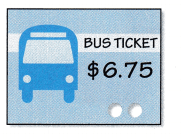He has one $5 bill. The below are the representation of coins$0.25 x 5 quarters + $0.10 x 5 dimes =$1.25 + $0.50 =$1.75